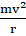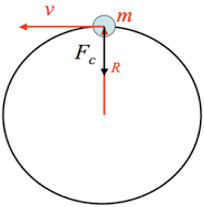### Question 3. What are (i) free fall, (ii) acceleration due to gravity (iii) escape velocity (iv) centripetal force?

Question 3.

Answer the following question.

What are (i) free fall, (ii) acceleration due to gravity (iii) escape velocity (iv) centripetal force?

Answer:

(i) Free Fall: Whenever an object moves under the influence of the force of gravity alone, it is said to be in Free Fall. During free fall initial velocity of an object is zero and force of air also acts on an object. Thus real free fall is possible only in a vacuum because there is no air.

For Example:

a) When an object is dropped from the top of the table it falls down only due to gravitational force hence it is under free fall.

b) An apple falling from the tree.

But when we are standing on the ground, flying in a flight we are not in free fall because other forces except gravitational force are also acting.

(ii) Acceleration due to gravity: The acceleration which is gained by an object because of the gravitational force is called acceleration due to gravity. Its S.I unit is m/s2. It is denoted by ‘g’ and its value at the surface of the earth is 9.8m/s2.

It is a vector quantity (have both magnitude and direction) and it is directed towards the centre of the earth. The value of acceleration due to gravity is not fixed it changes from place to place Like at moon ‘g’ value is one-sixth of value on earth.

(iii) Escape velocity: The minimum value of the initial velocity at which an object to escape from the gravitational pull of the earth and never comes back to the earth is called the escape velocity.

The escape velocity from earth is about 11.186 km/s, means if an object travels 11.186 km in 1 sec it will escape from earth’s gravitational pull. All the satellites and rockets are launched with a velocity equal to escape velocity in order to escape from the earth’s gravity.

(iv) Centripetal force: The force that acts on an object to keep it moving along the circular path with constant speed is called the centripetal force.

It is given by Fc =It is directed towards the centre of the circle (of radius ‘r’) in which the object is moving ( with velocity ‘v’).

Example: The stone tied to a piece of string whirl in a circle due to centripetal force only.

The diagram below illustrates the centripetal force acting on an object towards the centre.